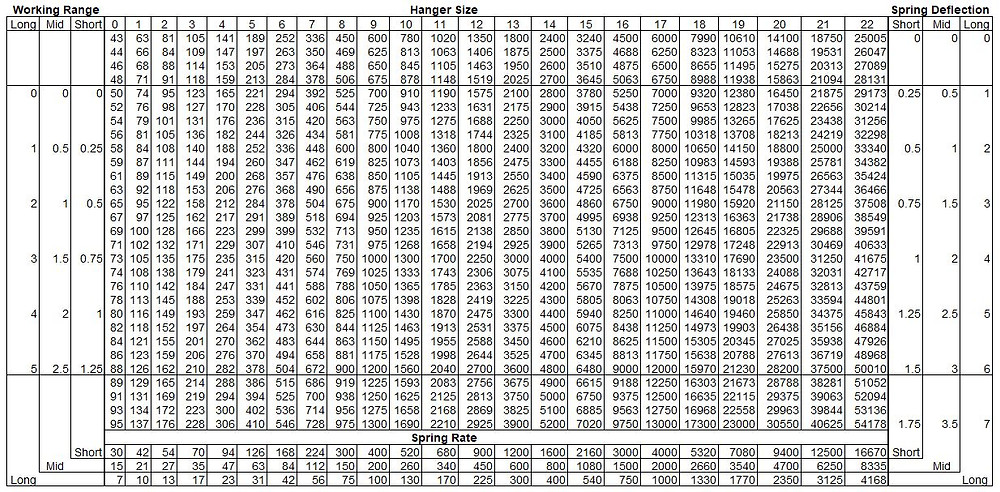Search

# 2.4.3 Hanger Selection Table

Springs are selected from a table such as that shown in Figure 2-31. This table shows the load capacities within the working ranges of each spring size, and the spring constants of the short-, mid-, and long-range spring series for each of the sizes. Knowing the hot load, thermal movement, and variability requirement, the process in selecting a spring from the table is:

1. Calculate the maximum permissible spring rate as: kmax = Var HL /I Δ th I

2. Determine the spring load size by finding the hot load in one of the columns of the hanger selection table.

3. For that size spring, select the spring series with a spring rate less than or equal to that calculated above.

4. Calculate the cold load (from CL = HL + k Δ th) and verify that the cold load also falls within the working range of the spring.

5. If this is not the case, then try again with a different spring series of the same size, or a spring of an adjacent size

This process may be illustrated by an example. Assume that the target hot load of a spring is computed as 613 pounds, the computed expected travel from cold to hot positions at that point is 1.5 inches up, the load variation limitation is 20%, and the spring manufacturer provides the selection table shown in Figure 2-31:

1. The maximum permissible spring rate is: kmax= 0.20x613/ I 1.5 I =82 lb/in

2. The hot load of 613 pounds is within the range of spring load size 7 (with a recommended range from 392 to 672 pounds), as shown in the selection table. Therefore, a spring of this size is a preliminary candidate for selection.

3. Looking at the bottom three lines of the column for size 7 shows that the only spring with a stiffness below the permissible spring rate is the long-range spring, with a stiffness of 56 lb/in.

4. For this spring, the Cold Load would be: CL = 613 + 56 x 1.5 = 697 lb. This load is outside of the recommended range of the spring (but does fall within the total range of 336 to 728 pounds, which should really only be used in extreme circumstances). Therefore, the size 7 spring should not be used.

5. It is now necessary to go through the calculation again, trying another spring. The hot load of 613 pounds also falls within the range of a size 8 spring (recommended range from 525 to 900 pounds), making this size also a candidate for selection. Looking at the spring rates for the size 8 spring, again only a long-range spring has a stiffness (75 lb/in) below the permissible rate. The Cold Load calculation is: CL = 613 + 75 x 1.5 = 726 lb. This load is within the recommended range of the spring; therefore, a long-range, size 8 spring pre-set to a Cold Load of 726 pounds must be specified.